Students can download 6th Science Term 1 Chapter 1 Measurements Questions and Answers, Notes, Samacheer Kalvi 6th Science Guide Pdf helps you to revise the complete Tamilnadu State Board New Syllabus, helps students complete homework assignments and to score high marks in board exams.

## Tamilnadu Samacheer Kalvi 6th Science Solutions Term 1 Chapter 1 Measurements

### Samacheer Kalvi 6th Science Measurements Text Book Back Questions and Answers

I. Choose the correct answer

Question 1.
The girth of a tree can be measured by
(a) Metre scale
(b) Metre rod
(c) plastic ruler
(d)Measuring tape.
(d) Measuring tape.

Question 2.
The conversion of 7 m into cm gives
(a) 70 cm
(b) 7 cm
(c) 700 cm
(d) 7000 cm
(c) 700 cmQuestion 3.
Quantity that can be measured is called ………
(a) Physical quantity
(b) Measurement
(c) Unit
(d) Motion.
(b) Measurement

Question 4.
Choose the correct one
(a) km > mm > cm > m
(b) km> mm> m > cm
(c) km>m>cm>mm
(d) km > cm > m > mm
(c) km > m > cm > mm

Question 5.
While measuring length of an oject using a ruler, the position of your eye should be
(a) Left side of the point.
(b) Vertically above the point where the measurement is to be taken.
(c) Right side of the point.
(d) Anywhere according to one’s convenience.
(b) Vertically above the point where the measurement is to be taken.

II. True or false.

1. We can say that mass an object is 126 kg
2. Length of one’s chest can be measured by using metre scale.
3. Ten millimetres makes one centimetre.
4. A hand span is a reliable measure of length.
5. The SI system of units is accepted everywhere in the world.

1. True
2. False
3. True
4. False
5. TrueIII. Fill up the blanks:

1. SI unit of length is symbolically represented as ………..
2. 500 gm = …………. kilogram.
3. Distance between Delhi and Chennai can be measured in ……….
4. 1 m = ………. cm.
5. 5 km = ………. m.

1. metre
2. 0.5
3. Kilometre
4. 100
5. 5000

IV. Analogy

Sugar: Beam Balance, Lime Juice?

Height of a person: Cm: Length of your sharpened pencil lead?
mm

Milk: Volume; vegetables?
Weight

V. Match the following: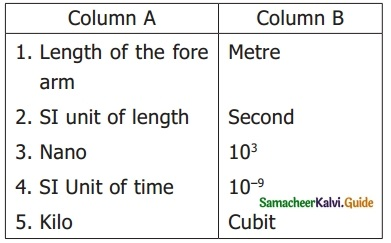1. Cubit
2. metre
3. 10-9
4. second
5. 10³VI. Complete the given table:VI. Arrange in increasing order of unit

1 Metre, 1 Centimetre, 1 Kilometre, and 1 Millimetre.
1 Millimetre, 1 Centimetre, 1 Metre, 1 Kilometre.

VIII. Find the answer for the following questions within the grid: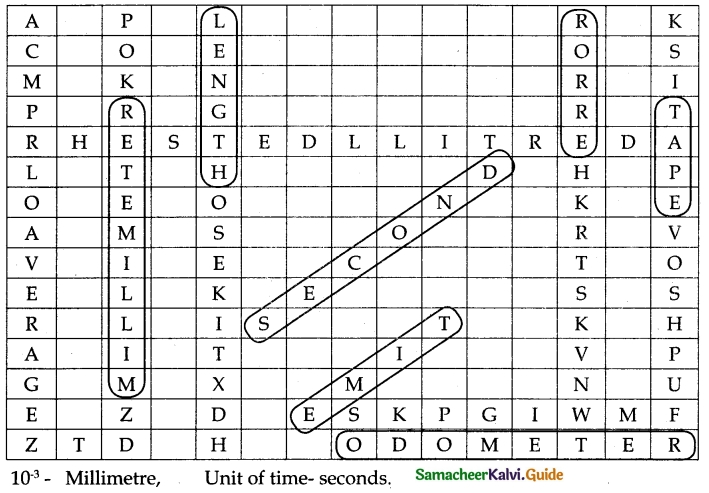1. 10-3 is one _______
2. SI unit of time ______
3. Cross view of reading for measurement leads to ______
4. _______ is the one what a clock reads.
5. ______ is the amount of substance present in an object.
6. _______ can be taken to get the final reading of the recordings of different students for a single measurement.
7. ______ is a fundamental quantity.
8. _______ shows the distance covered by an automobile.
9. A tailor use _______ to take measurements to stitch a cloth.
10. Liquids are measured with this physical quantity ______

1. millimeter
2. Second
3. Parallax
4. Time
5. mass
6. Accurate
7. Length
8. Odometer
9. tape
10. litresIX. Answer in a word or two.

Question 1.
What is the full form of the SI system?
An International System of unit

Question 2.
Name any one instrument used for measuring mass.
Beam Balance

Question 3.
Find the odd one out: Kilogram, Millimetre, Centimetre, Nanometre
Kilogram.

Question 4.
What is the SI unit of mass?
Kilogram

Question 5.
What are the two parts present in a measurement?

1. multiple
2. sub

X. Answer in a sentence or two:

Question 1.
Define measurement.
The comparison of an unknown quantity with some known quantity is known as measurement.

Question 2.
Define mass.
Mass is the measure of the amount of matter in an object.

Question 3.
The distance between the two places is 43.65 km. convert it into meter and cm.
(a) Convert km into metre
1 km = 1000m
∴ 43.65 km = 43.65 × 1000 = 43650.00 = 43650
= 43650 m.

(b) Convert km into cm.
1 km = 1000 m
1 m = 100 cm
1 km = 1000 × 100 cm
1 km = 100000 cm
∴ 43.65 km = 43.65 × 100000 = 4365000.00
= 4365000 cm.Question 4.
What are the rules to be followed to make an accurate measurement with scale?

1. Take care to write the correct submultiple.
2. Always keep the object in parallel to the scale.
3. Start the measurement from ‘0’ of the scale.

XI. Solve the following:

Question 1.
The distance between your school and your house is 2250 m. Express this distance in kilometre.
Distance between school and house is 2250 m.
1000 m = 1 km
∴ 2250 m = 2250 ÷ 1000 = 2.25 km.

Question 2.
While measuring the length of a sharpened pencil, the reading of the scale at one end is 2.0 cm and at the other end is 12.1 cm. What is the length of the pencil?
Sharpened pencil Reading at one end = 2.0 cm.
Sharpened pencil Reading at the other end = 12.1 cm.
Length of the pencil = Difference between two ends.
= 12.1 cm. – 2.0 cm.
= 10.1 cm.

XII. Write in detail:

Question 1.
Explain two methods that you can use to measure the length of a curved line.
Measuring the length of a curved line, by two methods.
First method – using a string.

1. Draw a curved line AB on the paper.
2. Place a string along the curved line.
3. Make sure that the string covers every bit of the curved line.
4. Mark the points where the curved line begins and ends on the string.
5. Now stretch the string along the length of a meter scale.
6. Measure the distance between two markings of the string.
7. This will give the length of a curved line.

Second method – using a divider.

1. Draw a curved line AB on a paper.
2. Separate the legs of the divider by 0.5 cm or 1 cm using a ruler.
3. Place it on the curved line starting from one end. Mark the position of the other end.
4. Move it along the line again and again cutting the line into a number of segments of equal lengths.
5. The remaining parts of the line can be measured using a scale.
6. Count the number of segments.
7. Length of the line = (No. of segments × length of each segment) + length of the leftover part.Question 2.
Fill up the following chart.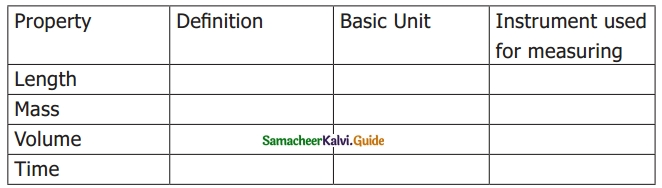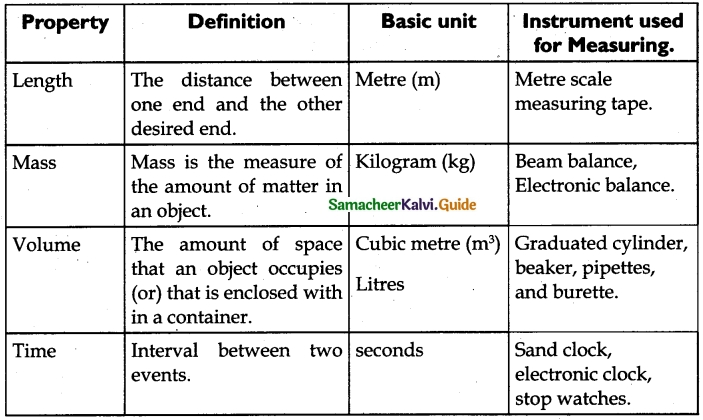### Samacheer Kalvi 6th Science Measurements Additional Important Questions and Answers

I. Choose the best option:

Question 1.
What is 7 m in mm?
(a) 0.7 mm
(b) 700 mm
(c) 7000 mm
(d) 70 mm
(c) 7000 mm

Question 2.
The distance between one end and the other end is called _____
(a) mass
(b) length
(c) time
(d) None
(b) length

Question 3.
What is the unit of length?
(a) metre
(b) litre
(c) second
(d) kilogram.
(a) metreQuestion 4.
Five kilometre is equal to _____
(a) 500 cm
(b) 500 m
(c) 5000 m
(d) 5000 cm
(c) 5000 m

Question 5.
These are the instruments used in measuring the volume of objects with irregular shapes.
(a) Balance
(b) Electronic balance
(c) Displacement of water method
(d) sand clock.
(c) Displacement of water method.

II. True or false:

1. The comparison of a known quantity with the unknown quantity is the mass.
2. The area can be calculated by using the two lengths and width.
3. Using electronic balance weight can be measured accurately.

1. False
2. True
3. False.

III. Fill in the Blanks.

1. 78.75 cm = ______ m ______ cm
2. 1195 m = ______km ______ m
3. 15 cm 10 mm = ______ mm
4. 45 km 33 m = ______ m.
5. The metric system of unit is developed in the year ______ by French.

1. -78 m, 75 cm
2. -1 km, 195 m
3. -160 mm
4. -45033 m
5. -1790IV. Matching

Question 1.1. – d
2. – c
3. – b
4. – a

Question 2.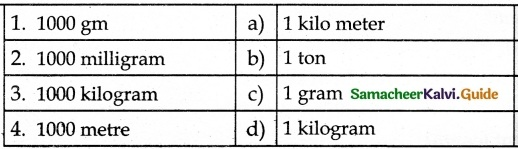1. – d
2. – c
3. – b
4. – a

V. Write the following unit in ascending order.

Question 1.
Ton – gram – kilogram – Metric ton
Gram – kilogram – ton – metric ton.

VI. Very Short Answer (2 marks)

Question 1.
What is the length?
The distance between one end and the other desired end is called length.

Question 2.
Differentiate mass from weight:
Mass :
Mass is the measure of the amount of matter in an object.
Unit – kilogram

Weight :
Weight is the gravitational pull experienced by the mass.
Unit – Newton.Question 3.
List the SI units of length, mass, and time.
Solution:

1. Length is measured by metre (m)
2. Mass is measured by the kilogram (kg)
3. Time is measured by second (s).

Question 4.
What is the basic quantity of physics?

1. Length
2. Mass
3. Time.

Question 5.
Differentiate of Mass and Weight.
Mass:

• Amount of matter in an object
• The measuring instrument is Beam balance

Weight:

• Gravitational pull experienced by the mass
• The measuring instrument is an electronic balance

Question 6.
What is the clock used to measure the time accurately?

1. Electronic clock
2. Stop clock

Question 7.
Hold a sheet paper in one hand and a book in one hand. Which hand feels heavy? Why?
Solution:
The hand, which holds the book, will feel heavy. The mass of the book is more than that of a single sheet of paper. Therefore, the pull on the book is more than that of the paper. Hence our hand has to give more force to hold a book than a paper.

VII. Give detailed answers: (5 marks)

Question 1.
What are the features that we must give importance in measuring?